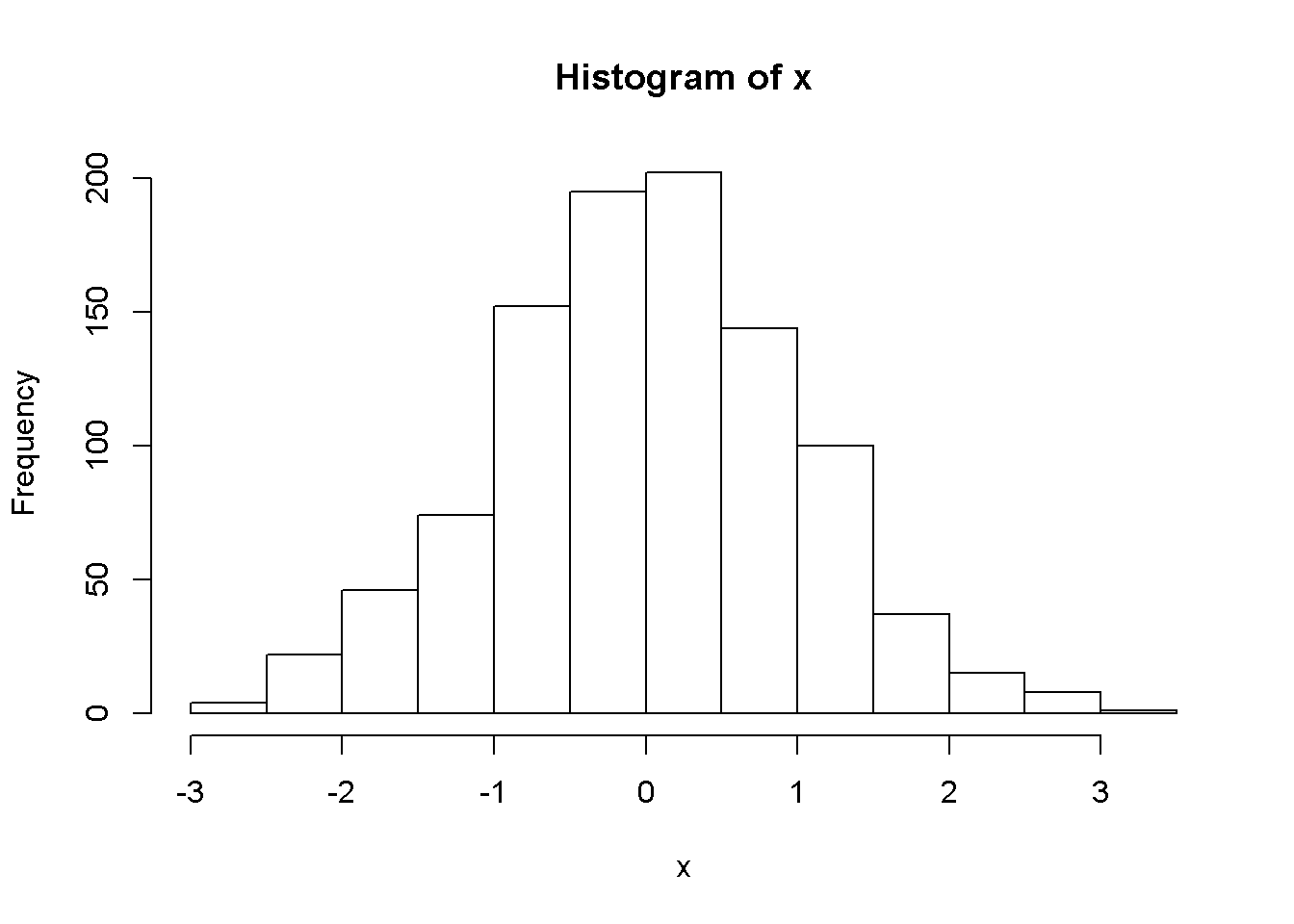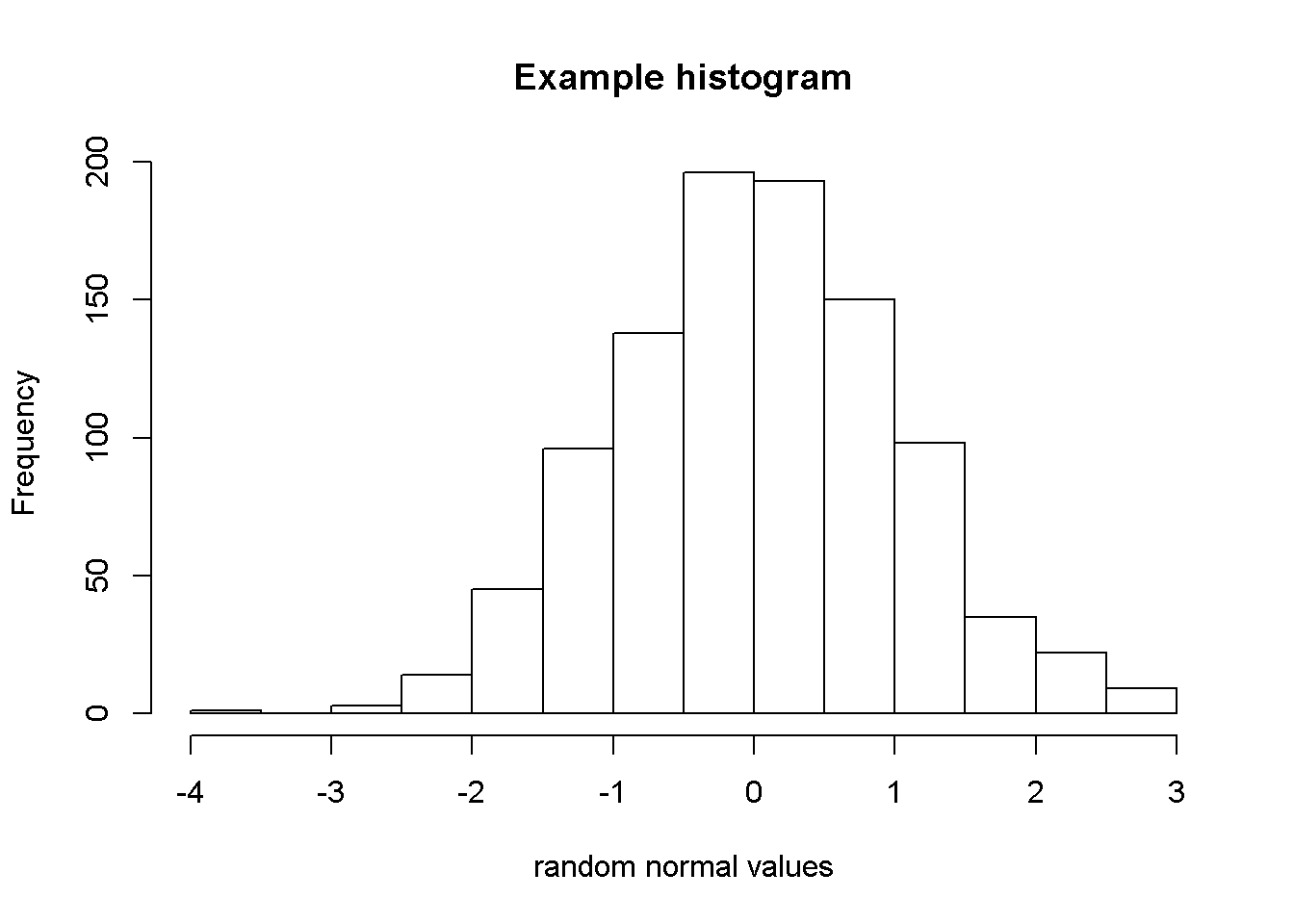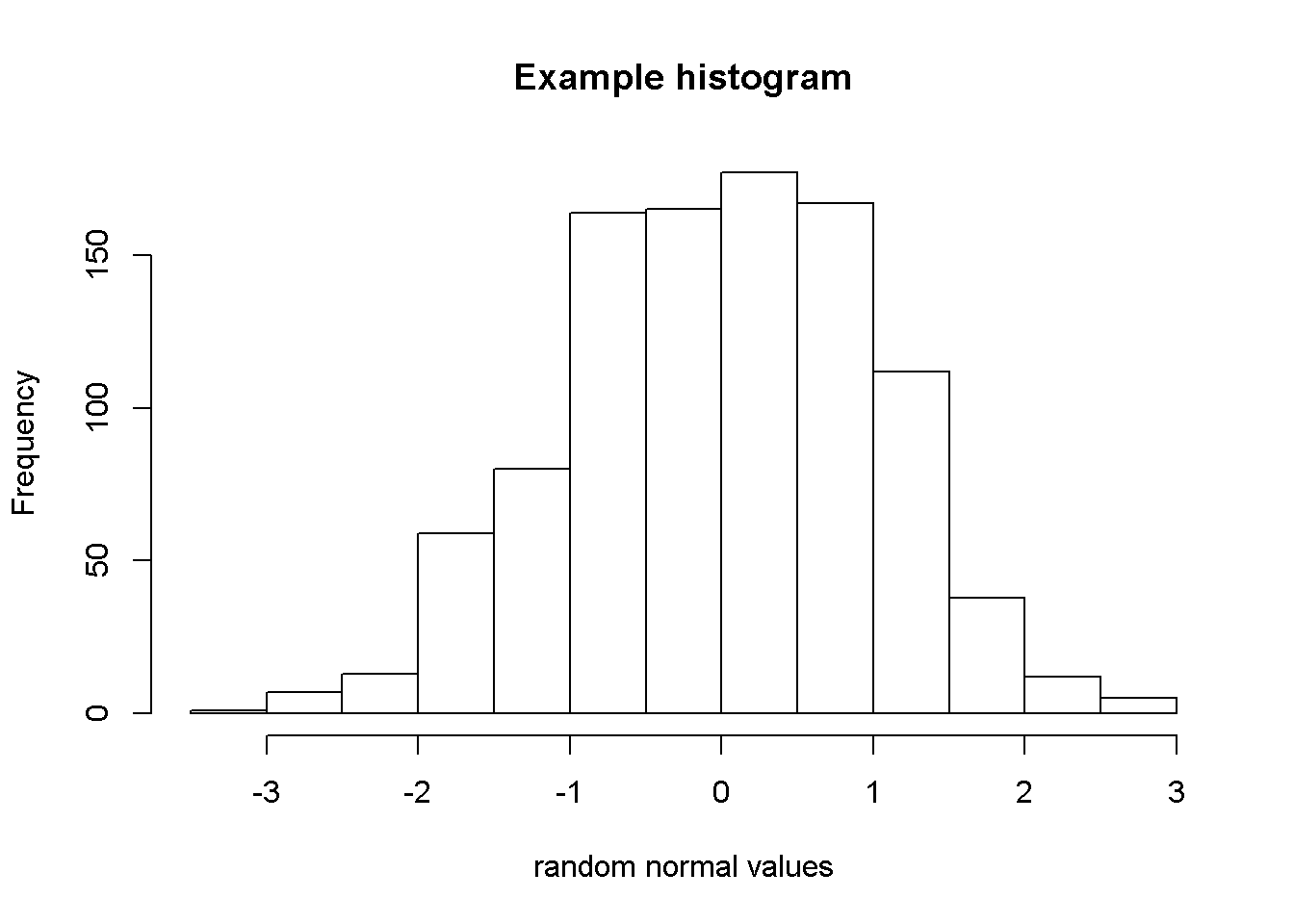# The BrailleR package Example 1.

## Histograms

The first and most commonly used example demonstrating the value of the BrailleR package to a blind user is the creation of a histogram.

x=rnorm(1000)
VI(hist(x))This is a histogram, with the title: Histogram of x
x is marked on the x-axis.
There are a total of 1000 elements for this variable.
It has 13 bins with equal widths, starting at -3 and ending at 3.5 .
The mids and counts for the bins are:
mid = -2.75  count = 4
mid = -2.25  count = 22
mid = -1.75  count = 46
mid = -1.25  count = 74
mid = -0.75  count = 152
mid = -0.25  count = 195
mid = 0.25  count = 202
mid = 0.75  count = 144
mid = 1.25  count = 100
mid = 1.75  count = 37
mid = 2.25  count = 15
mid = 2.75  count = 8
mid = 3.25  count = 1

The VI() command actually calls the VI.histogram() command as the hist() command creates an object of class “histogram”.

## Important features

The VI() command has added to the impact of issuing the hist() command as the actual graphic is generated for the sighted audience. The blind student can read from the text description so that they can interpret the information that the histogram offers the sighted world.

The above example showed the standard implementation of the hist() function. The hist() function of the graphics package does not store the additional arguments that improve the visual attractiveness. The solution (perhaps temporary) is to mask the original function with one included in the BrailleR package that calls the graphics package function, and then adds extra detail for any added plotting arguments.

This is best illustrated using the example included in the BrailleR::hist() function.

example(hist)

hist> # the stamdard hist function returns
hist> MyHist=graphics::hist(rnorm(1000), xlab="random normal values", main="Example histogram")hist> #dev.off()
hist> MyHist
$breaks  -4.0 -3.5 -3.0 -2.5 -2.0 -1.5 -1.0 -0.5 0.0 0.5 1.0 1.5 2.0 2.5  3.0$counts
   1   0   3  14  45  96 138 196 193 150  98  35  22   9

$density  0.002 0.000 0.006 0.028 0.090 0.192 0.276 0.392 0.386 0.300 0.196  0.070 0.044 0.018$mids
 -3.75 -3.25 -2.75 -2.25 -1.75 -1.25 -0.75 -0.25  0.25  0.75  1.25
  1.75  2.25  2.75

$xname  "rnorm(1000)"$equidist
 TRUE

attr(,"class")
 "histogram"

hist> # while this version returns
hist> MyHist=hist(rnorm(1000), xlab="random normal values", main="Example histogram")hist> #dev.off()
hist> MyHist
$breaks  -3.5 -3.0 -2.5 -2.0 -1.5 -1.0 -0.5 0.0 0.5 1.0 1.5 2.0 2.5 3.0$counts
   1   7  13  59  80 164 165 177 167 112  38  12   5

$density  0.002 0.014 0.026 0.118 0.160 0.328 0.330 0.354 0.334 0.224 0.076  0.024 0.010$mids
 -3.25 -2.75 -2.25 -1.75 -1.25 -0.75 -0.25  0.25  0.75  1.25  1.75
  2.25  2.75

$xname  "rnorm(1000)"$equidist
 TRUE

$main  "Example histogram"$xlab
 "random normal values"

attr(,"class")
 "histogram"

hist> # The VI() method uses the extra information stored
hist> VI(MyHist)
This is a histogram, with the title: Example histogram
random normal values is marked on the x-axis.
There are a total of 1000 elements for this variable.
It has 13 bins with equal widths, starting at -3.5 and ending at 3 .
The mids and counts for the bins are:
mid = -3.25  count = 1
mid = -2.75  count = 7
mid = -2.25  count = 13
mid = -1.75  count = 59
mid = -1.25  count = 80
mid = -0.75  count = 164
mid = -0.25  count = 165
mid = 0.25  count = 177
mid = 0.75  count = 167
mid = 1.25  count = 112
mid = 1.75  count = 38
mid = 2.25  count = 12
mid = 2.75  count = 5

## Warning

The VI() function is partially reliant on the use of the hist() function that is included in the BrailleR package. If a histogram is created using a command that directly links to the original hist() command found in the graphics package, then the VI() command’s output will not be as useful to the blind user. This mainly affects the presentation of the title and axis labels; it should not affect the details of the counts etc. within the histogram itself.

This behaviour could arise if the histogram is sought indirectly. If for example, a function offers (as a side effect) to create a histogram, the author of the function may have explicitly stated use of the hist() function from the graphics package using graphics::hist() instead of hist(). Use of graphics::hist() will bypass the BrailleR::hist() function that the VI() command needs. This should not create error messages.